# Trigonometric Fourier Series

We have already discussed the Fourier series in exponential form. In this article we will discuss another form of Fourier series i.e. Trigonometric Fourier series.

## Fourier series representation in Trigonometric form

Fourier series in trigonometric form can be easily derived from its exponential form. The complex exponential Fourier series representation of a periodic signal x(t) with fundamental period To is given by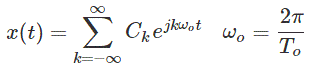Since sine and cosine can be expressed in exponential form. Thus by manipulating the exponential Fourier series, we can obtain its Trigonometric form.

The trigonometric Fourier series representation of a periodic signal x (t) with fundamental period T, is given by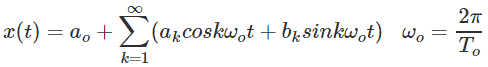Where ak and bk are Fourier coefficients given by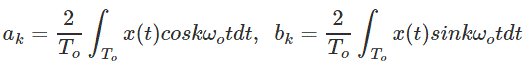a0 is the dc component of the signal and is given by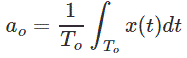## Properties of Fourier series

1. If x(t) is an even function i.e. x(- t) = x(t), then bk = 0 and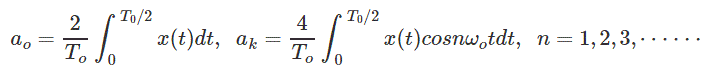2. If x(t) is an even function i.e. x(- t) = – x(t), then a0 = 0, ak = 0 and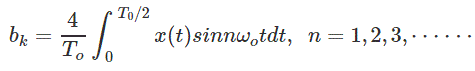3. If x(t) is half symmetric function i.e. x (t) = -x(t ± T0/2), then a0 = 0, ak = bk = 0 for k even,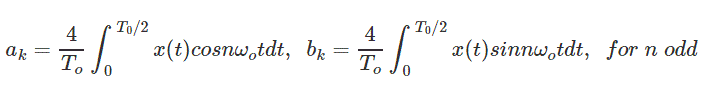4. Linearity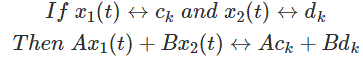5. Time shifting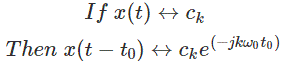6. Time reversal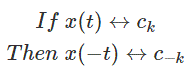7. Multiplication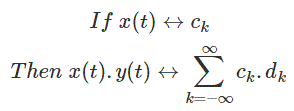8. Conjugation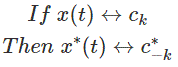9. Differentiation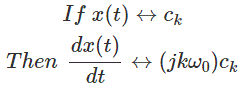10. Integration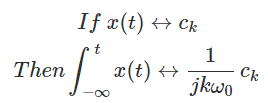11. Periodic Convolution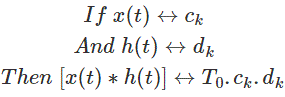#### Relationship between coefficients of exponential form and coefficients of trigonometric form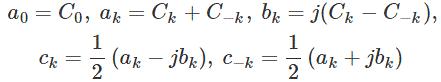When x (t) is real, then a, and b, are real, we have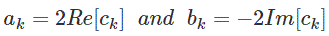### Effect of Shifting Axis of the Signal

• On shifting the waveform to the left right with respect to the reference time axis t = 0 only the phase values of the spectrum changes but the magnitude spectrum remains same.
• On shifting the waveform upward or downward w.r.t time axis changes only the DC value of the function.

Want To Learn Faster? 🎓
Get electrical articles delivered to your inbox every week.
No credit card required—it’s 100% free.